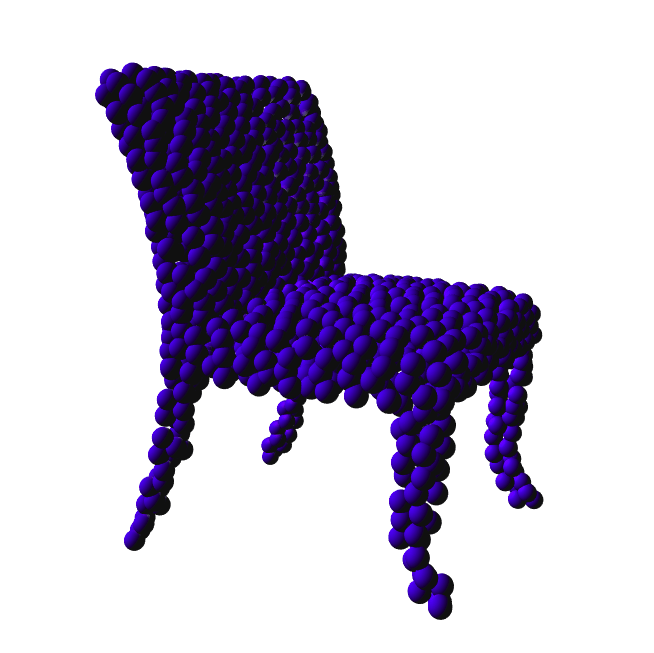# PointNet Classification

Classification of PointCloud structure using PointNet model

Note

For visualization purpose we will require to install Makie and compatible backend (GLMakie or WGLMakie). To install it simply run ] add Makie in the julia prompt.

using Flux3D, Flux, Makie, CUDA
using Flux: onehotbatch, onecold, onehot, crossentropy
using Statistics: mean
using Base.Iterators: partition

Makie.inline!(false)
Makie.set_theme!(show_axis = false)

## Defining arguments for use in training.

• batch_size - batch size of of training data to be passed while training.
• lr - learing rate for the optimization.
• epochs - number of episodes for training the classification model.
• num_classes - number of classes in labels of dataset.
• npoints - number of points in each PointCloud to be returned by dataset.
batch_size = 32
lr = 3e-4
epochs = 5
num_classes = 10 #possible values {10,40}
npoints = 1024

## ModelNet10 Dataset

This package has dataset wrapper for ModelNet10/40 which makes it easy to load and preprocess ModelNet dataset. In this example we will using ModelNet10 but we can also use ModelNet40 with minor tweak in num_classes args.

We can construct ModelNet10 dataset by passing:

• mode=:pointcloud - for returning PointCloud variant of dataset
• npoints - no. of points in each PointCloud.
• transforms - Transforms to be applied before return specified PointCloud.
• train - Bool to indicate training or testing split.

Detailed list of available arguments can be found in ModelNet section.

dset = ModelNet10.dataset(;
mode = :pointcloud,
npoints = npoints,
transform = NormalizePointCloud(),
)
val_dset = ModelNet10.dataset(;
mode = :pointcloud,
train = false,
npoints = npoints,
transform = NormalizePointCloud(),
)

## Visualizing the dataset

we can access the dataset by correspond index like dset which will return a ModelNet10 DataPoint and following information idx: 1, data: PointCloud{Float32}, ground_truth: 1 (bathtub).

For the visulizing the corresponding datapoint we can use visulize

visualize(dset, markersize = 0.1)data = [dset[i].data.points for i = 1:length(dset)]
labels =
onehotbatch([dset[i].ground_truth for i = 1:length(dset)], 1:num_classes)

valX = cat([val_dset[i].data.points for i = 1:length(val_dset)]..., dims = 3)
valY = onehotbatch(
[val_dset[i].ground_truth for i = 1:length(val_dset)],
1:num_classes,
)

TRAIN = [
(cat(data[i]..., dims = 3), labels[:, i])
for i in partition(1:length(data), batch_size)
]
VAL = (valX, valY)

## Defining 3D model

Flux3D has predefined PointNet classification model which can be used to train PointCloud dataset

m = PointNet(num_classes)

## Defining loss and validating objectives

loss(x, y) = crossentropy(m(x), y)
accuracy(x, y) =
mean(onecold(cpu(m(x)), 1:num_classes) .== onecold(cpu(y), 1:num_classes))

## Defining learning rate and optimizer

opt = Flux.ADAM(lr)

## Using GPU for fast training [Optional]

We can convert the 3D model to GPU or CPU usinggpu and cpu, and also changing the dataloader using same function

m = m |> gpu
TRAIN = TRAIN |> gpu
VAL = VAL |> gpu

## Training the 3D model

ps = params(m)
for epoch = 1:epochs
running_loss = 0
for d in TRAIN
print("Epoch: $(epoch), epoch_loss:$(running_loss), accuracy: \$(accuracy(VAL...))\n")
@show accuracy(VAL...)